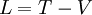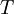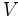# Lagrangian

The Lagrangian of a dynamical system is a function used to describe the dynamics of that system. In classical mechanics, the general definition of the Lagrangian is a function used to describe the difference between the kinetic and potential energies of a dynamical system:whereis the kinetic energy andis the potential energy.

The Lagrangian of a system can be used in the Euler-Lagrange equation in order to derive the equations of motion for the system.

The general idea behind the Lagrangian is to divorce the dynamical system from any specific coordinate system.